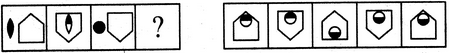Non Verbal Reasoning - Analogy - Discussion

Discussion :: Analogy - Section 1 (Q.No.15)

Each of the following questions consists of two sets of figures. Figures A, B, C and D constitute the Problem Set while figures 1, 2, 3, 4 and 5 constitute the Answer Set. There is a definite relationship between figures A and B. Establish a similar relationship between figures C and D by selecting a suitable figure from the Answer Set that would replace the question mark (?) in fig. (D).

15.

Select a suitable figure from the Answer Figures that would replace the question mark (?).

Problem Figures:                            Answer Figures:(A)     (B)      (C)     (D)                  (1)      (2)      (3)      (4)      (5)

 [A]. 1 [B]. 2 [C]. 3 [D]. 4 [E]. 5

Explanation:

The pentagon gets vertically inverted. The lower half of the black element becomes white and this element moves inside the pentagon and gets attached to its upper end.

 Nick said: (Sep 27, 2013) This could also be explained by: The pentagon gets vertically inverted. The lower half of the black element becomes white and this element moves inside the pentagon and gets attached to the base of the pentagon - but this would give an answer of number (3) or C.

 Eram said: (Feb 17, 2015) I think C is the answer because pentagon will get vertically inverted and the upper half will become white as related to figure B.

 Jady said: (Jul 13, 2015) I think C should be right as well. The element gets attached to the bottom.

 Mr.Bit said: (May 4, 2016) I agree with you all. The answer should be option C.

 Shubham Thakur said: (Sep 27, 2017) I think C is the correct one.

 Akshay said: (Jul 10, 2019) I think it's C.